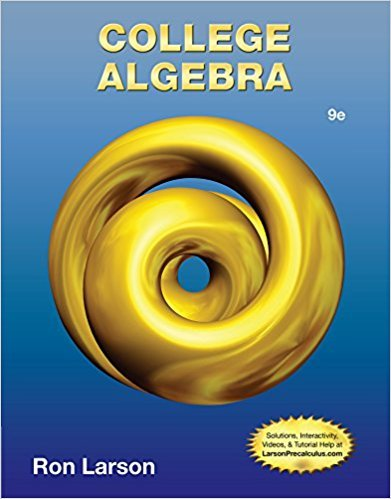×
Log in to StudySoup
Get Full Access to College Algebra - 9 Edition - Chapter 7 - Problem 7.1.2
Join StudySoup for FREE
Get Full Access to College Algebra - 9 Edition - Chapter 7 - Problem 7.1.2

Already have an account? Login here
×
Reset your password

# A matrix is ________ when the number of rows equals theISBN: 9781133963028 204

## Solution for problem 7.1.2 Chapter 7

College Algebra | 9th Edition

• Textbook Solutions
• 2901 Step-by-step solutions solved by professors and subject experts
• Get 24/7 help from StudySoup virtual teaching assistantsCollege Algebra | 9th Edition

4 5 1 320 Reviews
25
2
Problem 7.1.2

A matrix is ________ when the number of rows equals the number of columns.

Step-by-Step Solution:
Step 1 of 3

▯▯▯▯▯▯▯▯▯▯▯▯▯▯▯▯▯▯▯▯▯ ▯▯▯▯▯▯▯▯▯▯▯▯▯▯▯▯▯▯▯▯▯▯▯▯▯▯▯ ▯▯▯▯▯▯▯ ▯▯▯▯▯▯▯▯▯▯▯▯▯▯▯▯▯▯▯▯▯▯▯▯▯▯▯ ▯▯▯▯▯▯▯ ▯▯▯▯▯▯▯▯▯▯▯▯▯▯▯▯▯▯▯▯▯▯▯▯▯▯▯ ▯▯▯▯▯▯▯ ▯▯▯▯▯▯▯▯▯▯▯▯▯▯▯▯▯▯▯▯▯▯▯▯▯▯▯ ▯▯▯▯▯▯▯ ▯▯▯▯▯▯▯▯▯▯▯▯▯▯▯▯▯▯▯▯▯▯▯▯▯▯▯ ▯▯▯▯▯▯▯

Step 2 of 3

Step 3 of 3

##### ISBN: 9781133963028

College Algebra was written by and is associated to the ISBN: 9781133963028. This textbook survival guide was created for the textbook: College Algebra, edition: 9. Since the solution to 7.1.2 from 7 chapter was answered, more than 336 students have viewed the full step-by-step answer. This full solution covers the following key subjects: . This expansive textbook survival guide covers 9 chapters, and 5750 solutions. The answer to “A matrix is ________ when the number of rows equals the number of columns.” is broken down into a number of easy to follow steps, and 14 words. The full step-by-step solution to problem: 7.1.2 from chapter: 7 was answered by , our top Math solution expert on 01/02/18, 09:21PM.

Unlock Textbook Solution

Enter your email below to unlock your verified solution to:

A matrix is ________ when the number of rows equals the